AP Chemistry : Phase Changes

Example Questions

← Previous 1 3

Example Question #1 : Energy Of Phase Changes

As condensation forms on a glass of ice water, the temperature of the air surrounding the glass __________.

increases

stays the same

decreases

is impossible to determine

increases

Explanation:

As a substance condenses from the gas phase to the liquid phase, it loses energy in the form of heat loss. Heat is transferred from the water to the air, resulting in an increase in the temperature of the air.

Example Question #2 : Energy Of Phase Changes

How much energy is required to boil 9 moles of liquid water at its boiling point, and what is the temperature of the water vapor product?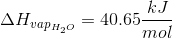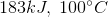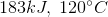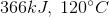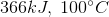Explanation:

The enthalpy of vaporization gives the amount of energy required to evaporate a liquid at its boiling point, in units of energy per mole. The total energy requirement to heat a given amount of steam is found by mulitplying the the number of moles to be vaporized by the energy of vaporization per mole.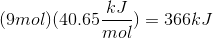The temperature remains constant throughout a phase change, thus the final temperature would still be 100°C.

Example Question #3 : Energy Of Phase Changes

How much heat must be added to raise a sample of 100g of water at 270K to 280K?

The specific heat capacity of water is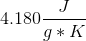, and water's heat of fusion is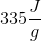.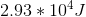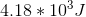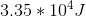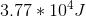Explanation:

The following fomula gives the heat needed to generate a given temperature change for a substance of known specific heat capacity: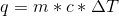where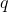is the heat input in Joules,is the mass of the sample in grams, andis the specific heat capacity in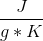.

However, in the event of a phase change (water melts at 273K), the heat of fusion or vaporization must be added to the total energy cost. The formula becomes: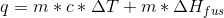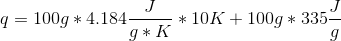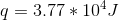Example Question #4 : Energy Of Phase Changes

Why does water boil at a lower temperature at high elevation?

The atmospheric pressure is lower at high elevations

Water has a higher vapor pressure at high elevation

There is a lower heat of fusion at higher elevation

Hydrogen bonds are easier to disrupt at high elevation

The higher the elevation, the denser water is.

The atmospheric pressure is lower at high elevations

Explanation:

Increasing temperature means that vapor pressure increases as well. When vapor pressure is equal to the atmospheric pressure, water boils. The atmospheric pressure is lower at high elevation, so water boils at a lower temperature.

Example Question #5 : Energy Of Phase Changes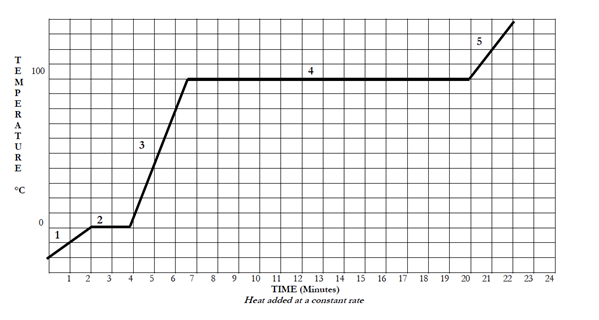Using the heat curve, define the segment time(s) that the kinetic energy of the substance is increasing.

1, 3, and 5

2 and 4

2, 3, and 4

1, 2, and 5

1, 3, and 5

Explanation:

Remember, temperature is a measure of the average kinetic energy of molecules. Therefore the kinetic energy increases whenever the temperature is increasing. So, the kinetic energy is increasing during segments 1, 3, and 5.

Example Question #6 : Energy Of Phase ChangesUsing the heating curve, determine which segment(s) relate to an increase in potential energy.

2, 3, and 4

1, 2, 3, 4, and 5

1, 3, and 5

2 and 4

2 and 4

Explanation:

Remember, temperature is a measure of the average kinetic energy of molecules. So, the potential energy of the molecules will increase anytime energy is being supplied to the system but the temperature is not increasing. Therefore the potential energy is increasing during segments 2 and 4.

Example Question #7 : Energy Of Phase ChangesThe heating curve represents a substance in phases solid, liquid, and gas. Which segment represents only the liquid phase?

4

1

2

3

3

Explanation:

When the kinetic energy is increasing (the temperature is also increasing) the substance is not going through a phase change. Therefore only the segments that are at an incline will have the substance in just one phase. The flat areas of the graph represent areas in which heat is being added, but there is no corresponding increase in temperature. Rather, this added heat energy is used to break the intermolecular forces between molecules/atoms and drive phase changes.

Finally, because liquids are higher in energy than solids, and lower in energy than gasses the middle slanted line must be the liquid phase. In this case it is labeled as segment 3.

Example Question #8 : Energy Of Phase ChangesIn the given heating curve, which segment(s) correlate to a mixture of phases?

2, 4

1, 2, 3, 4, 5

1, 3, 5

2, 3, 4

2, 4

Explanation:

When kinetic energy is increasing molecules are simply moving faster. However, when the potential energy is increasing molecules are changing phases. Therefore, when the potential energy is increasing is when the molecule is changing phases. Therefore there is a mix of molecules during segments 2 and 4.

Example Question #9 : Energy Of Phase ChangesThe given heating curve represents a substance in phases solid, liquid, and gas. Which segment represents the substance as it is boiling?

2

4

3

5

4

Explanation:

Boiling is a phase change from liquids to gas. Therefore we are looking for a segment that is flat (because the potential energy is increasing) and that is between the liquid and gas phases.

In this case, gas phase is the highest energy phase, and liquids is the next highest. Therefore the substance is boiling during segment 4.

Example Question #10 : Energy Of Phase ChangesIn the heating curve shown above, at what point do the molecules have the highest kinetic energy?

The end of segment 1

The end of segment 5

The beginning of segment 1

The beginning of segment 5